In :
# Downloading all the data
!wget https://datasets.aicrowd.com/default/aicrowd-public-datasets/seamai-facies-challenge/v0.1/public/data_train.npz
!wget https://datasets.aicrowd.com/default/aicrowd-public-datasets/seamai-facies-challenge/v0.1/public/labels_train.npz
!wget https://datasets.aicrowd.com/default/aicrowd-public-datasets/seamai-facies-challenge/v0.1/public/data_test_1.npz

In :
# Get the seismiQB library with all the dependencies
!pip install segyio

!git clone --recursive -b improvements https://github.com/gazprom-neft/seismiqb.git

In :
# All the necessary imports
import sys
import os

import numpy as np
import matplotlib.pyplot as plt
import cv2

sys.path.insert(0, '/content/seismiqb')
from seismiqb import plot_image, Horizon, HorizonMetrics, SeismicGeometry

In :
CLASS_LABELS = [
'Basement/other',
'Slope Mudstone A',
'Mass Transport\n Deposit',
'Slope Mudstone B',
'Slope Valley',
'Submarine Canyon\n System'
]

In :
%%time
train_geometry = SeismicGeometry('/content/data_train.npz', order=(2, 1, 0))
train_labels = SeismicGeometry('/content/labels_train.npz', order=(2, 1, 0))

test_geometry = SeismicGeometry('/content/data_test_1.npz', order=(2, 1, 0))

CPU times: user 26.6 s, sys: 1.99 s, total: 28.6 s
Wall time: 28.7 s

In :
train_data_array = train_geometry.data['data']
train_labels_array = train_labels.data['labels']

test_data_array = test_geometry.data['data']

In :
# A simple test that everything is fine
plot_image(train_data_array[100, :, :], cmap='gray', figsize=(8, 8))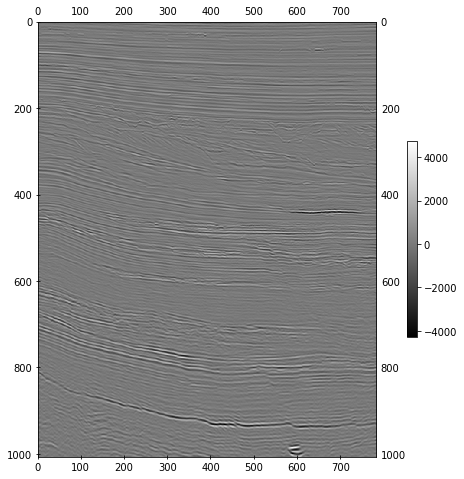# Amplitudes EDA¶

The first thing we can look at is the raw amplitude values inside the cube. As we will see later, we can augment them by applying some geological modifications (also known as attributes), but for now, let's focus on the values itself.

In :
%%time
mean, std = train_data_array.mean(), train_data_array.std()

plt.figure(figsize=(8, 5))
plt.hist(train_data_array.flatten(), bins=100)

plt.axvline(mean, color='k', linestyle='dashed', linewidth=2)
plt.axvline(mean + 3*std, color='k', linestyle='dashed', linewidth=1)
plt.axvline(mean - 3*std, color='k', linestyle='dashed', linewidth=1)
plt.show()

plt.figure(figsize=(8, 5))
plt.hist(train_data_array.flatten(), bins=100, log=True)

plt.axvline(mean, color='k', linestyle='dashed', linewidth=2)
plt.axvline(mean + 3*std, color='k', linestyle='dashed', linewidth=1)
plt.axvline(mean - 3*std, color='k', linestyle='dashed', linewidth=1)
plt.show()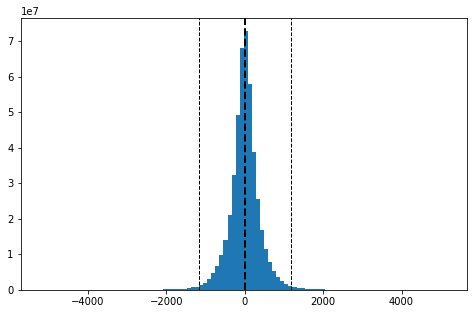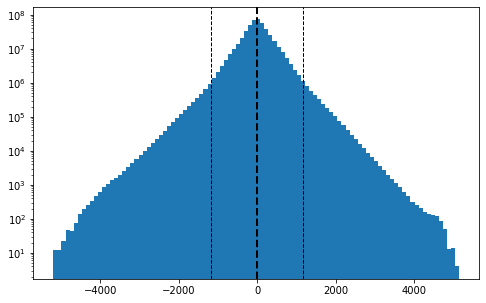CPU times: user 14.7 s, sys: 2.02 s, total: 16.7 s
Wall time: 16.2 s


From the shape of histograms we can see that amplitudes are distributed normally, and that we can use a simple quantile scaling for normalizing our data.

In :
%%time
ranges = train_data_array.min(), train_data_array.max()
values = np.unique(train_labels_array)

data_histograms = [train_data_array[train_labels_array == value].flatten()
for value in values]

ranges, values

CPU times: user 9.9 s, sys: 2.51 s, total: 12.4 s
Wall time: 12.4 s

Out:
((-5195.5234, 5151.7188), array([1, 2, 3, 4, 5, 6], dtype=int8))

We can look at distributions of amplitudes along each label:

In :
plt.figure(figsize=(12, 8))

for i, value in enumerate(values):
data = data_histograms[i]

label_name = CLASS_LABELS[value-1].replace('\n', '')
plt.hist(data, log=True, bins=50, range=ranges,
label=f'value {value}: {label_name}', alpha=0.2)

plt.legend(loc='best')
plt.show()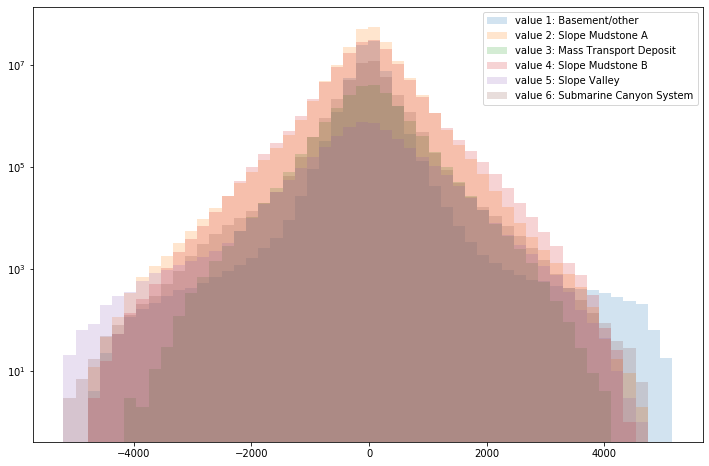It is hard to see anything from the previous figure. By plotting each of them on separate axes, we can easily see that label 1 and 5 are very different from all the rest: that can be used to improve our prediction scores!

In :
fig, ax = plt.subplots(3, 2, figsize=(14, 10))

for i, value in enumerate(values):
data = data_histograms[i]
mean_, std_ = data.mean(), data.std()
ax_ = ax[i // 2, i % 2]

label_name = CLASS_LABELS[value-1].replace('\n', '')

ax_.hist(data, log=True, bins=50, range=ranges, color='b',
label=f'value {value}: {label_name}', alpha=0.2)
ax_.axvline(mean_, color='k', linestyle='dashed', linewidth=2)
ax_.axvline(mean_ + 3*std_, color='k', linestyle='dashed', linewidth=1)
ax_.axvline(mean_ - 3*std_, color='k', linestyle='dashed', linewidth=1)

ax_.legend(loc='best')

print(f'{value} ::: mean is {mean_:4.4} ::: std is {std_:4.4} ::: {label_name}')

fig.show()

1 ::: mean is -0.8623 ::: std is 213.2 ::: Basement/other
2 ::: mean is 0.9905 ::: std is 379.5 ::: Slope Mudstone A
3 ::: mean is -5.073 ::: std is 468.9 ::: Mass Transport Deposit
4 ::: mean is 2.11 ::: std is 453.0 ::: Slope Mudstone B
5 ::: mean is -18.73 ::: std is 655.1 ::: Slope Valley
6 ::: mean is 1.998 ::: std is 372.3 ::: Submarine Canyon System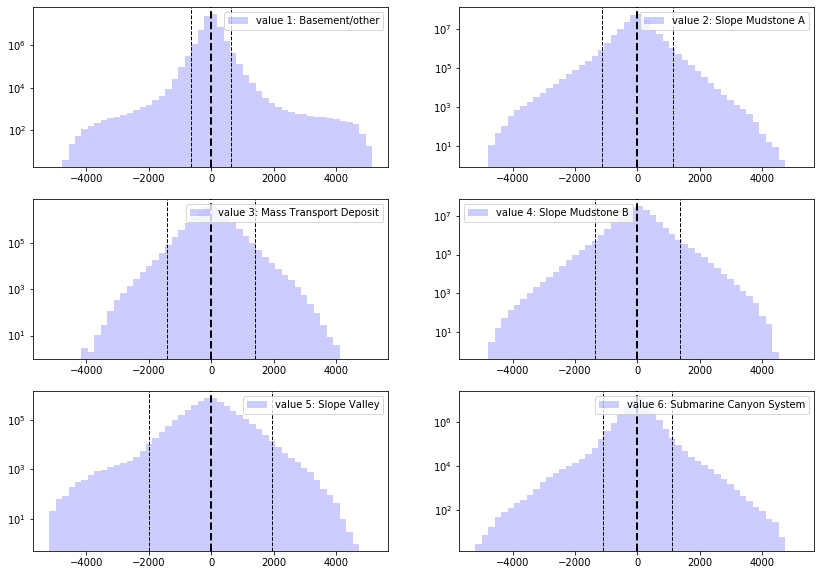# Signal-to-noise¶

An easy way to look at the cube as a whole is to compute signal-to-noise ratio along the depth axis:

In :
def plot_snr(array, **kwargs):
mean_matrix = np.mean(array, axis=-1)
std_matrix = np.std(array, axis=-1)
snr = np.log(mean_matrix**2 / std_matrix ** 2)
plot_image(snr, figsize=(8, 5), **kwargs)

In :
plot_snr(train_data_array, title='SNR train')

plot_snr(test_data_array, title='SNR test')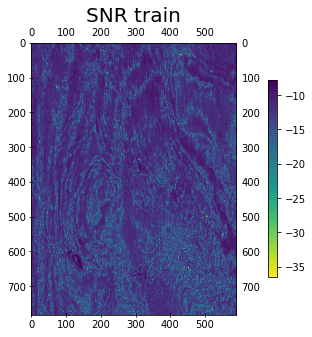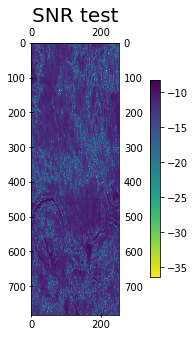We can easily see that the test part adjoins the training part from the right side. Overall, the cube is quite homogeneous and does not contain significant noises or signal losses.

# Depth analysis¶

It can be seen that labels are distributed differently along the depth-axis, and one can certainly use that to get better scores. Let's look at those distributions more closely:

In :
%%time
ranges = 0, train_labels_array.shape

data_depths = [np.nonzero(train_labels_array == value)
for value in values]

CPU times: user 9.23 s, sys: 4.3 s, total: 13.5 s
Wall time: 13.5 s

In :
plt.figure(figsize=(14, 8))

for i, value in enumerate(values):
data = data_depths[i]
label_name = CLASS_LABELS[value-1].replace('\n', '')

plt.hist(data, bins=150, range=ranges, orientation='horizontal',
label=f'value {value}: {label_name}', alpha=0.2)

plt.gca().invert_yaxis()
plt.legend(loc='best')
plt.show()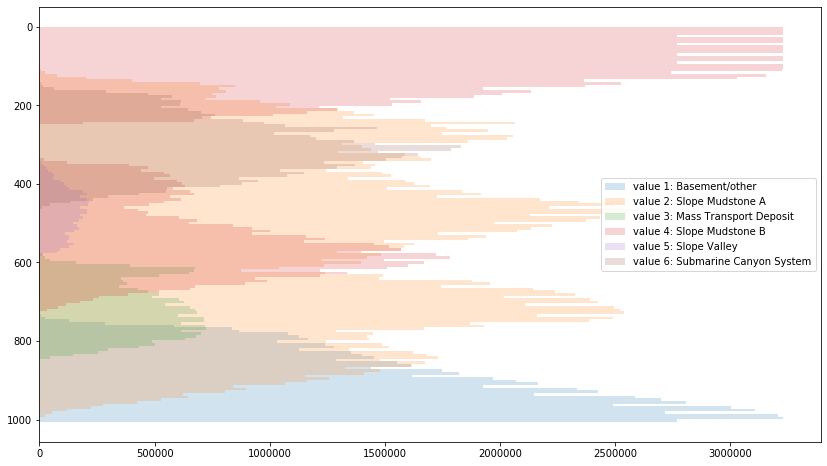We can see that some of the classes are overlapping, while some do not. Also, one of the classes has very small support: it can be a good idea to train a separate model for that class to improve the final score!

# Horizons analysis¶

You can see a lot of distinct and clearly visible lines on the seismic images above. These are seismic horizons -- reflectors between different types of rocks. They are essential in structural analysis of such data, as any change in subterranean field should also change the rock properties, thus producing a horizon.

The boundary of each of the tracked facies should be along some horizon. Luckily, we can extracy those horizons with ease be virtue of taking a difference of labels array along the DEPTH axis. Then, we can evaluate the quality of those horizons, and/or use them for training: it is obvious, that the task of segmenting 3D bodies can be seen as segmenting 2D boundaries between them!

In :
plot_image(train_labels_array[100, :, :], cmap='Accent')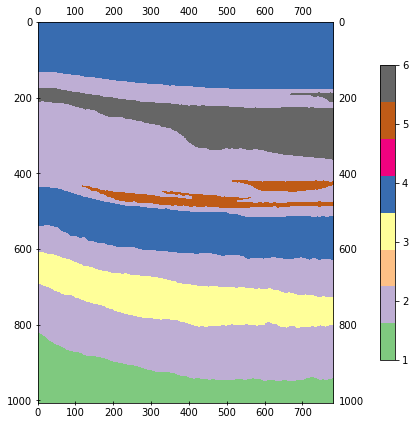By taking the difference of subsequent values along depth axis, we can extract boundaries. They are exactly horizons we seek:

In :
diff = np.diff(train_labels_array, prepend=0, axis=-1)
plot_image(diff[100, :, :], cmap='gray')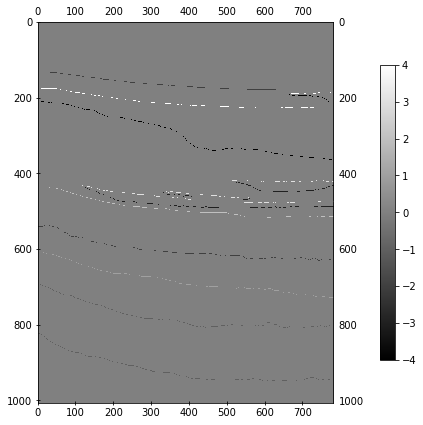In :
%%time
values = np.unique(diff, return_counts=True)
print(values)

values = values.tolist()
values.remove(0)
values

(array([-4, -3, -2, -1,  0,  1,  2,  3,  4]), array([   616502,    321679,    922744,    858841, 459167700,    401125,
460718,    321691,   1077280]))
CPU times: user 12.3 s, sys: 1.57 s, total: 13.9 s
Wall time: 13.8 s

Out:
[-4, -3, -2, -1, 1, 2, 3, 4]

Horizon.from_mask performs some magic to actually extract surfaces from the 3D array of differences. We will look at them more closely later.

In :
%%time
horizons_list = []

for value in tqdm(values):

structure = np.ones((3, 3), dtype=np.uint8)

minsize=10000, mode='max')
horizons_list.extend(horizons)

print(f'total horizons on value {value}: {len(horizons)}')
print('Lengths of horisons: ', *[len(item) for item in horizons], '\n')

horizons_list.sort(key=lambda item: item.h_mean)

total horizons on value -4: 3
Lengths of horisons:  12386 143309 461380

total horizons on value -3: 4
Lengths of horisons:  3051 8095 80622 228186

total horizons on value -2: 2
Lengths of horisons:  460826 461380

total horizons on value -1: 2
Lengths of horisons:  157448 460668

total horizons on value 1: 2
Lengths of horisons:  157448 243050

total horizons on value 2: 1
Lengths of horisons:  460826

total horizons on value 3: 2
Lengths of horisons:  80622 228186

total horizons on value 4: 4
Lengths of horisons:  12386 143309 461380 461380

CPU times: user 1min 3s, sys: 33.6 s, total: 1min 36s
Wall time: 1min 36s


Now we want to remove some of the horizons to work only with the biggest ones.

In :
horizons = []

for horizon in horizons_list:
if abs(horizon.h_mean - already_stored.h_mean) < 4.:
break
else:
horizons.append(horizon)

horizons = [item for item in horizons
if len(item) > 100000 and item.h_std > 1. and (item.full_matrix[500:, :] != Horizon.FILL_VALUE).sum() > 40000]

horizons.sort(key=len)

len(horizons_list), len(horizons)

Out:
(20, 7)

We can look at the resulting surface from above. It is essentialy a map of depth for that horizon:

In :
horizons[-1].show()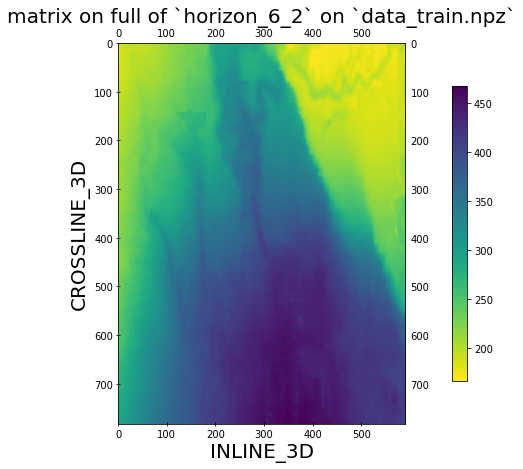One would need a touch of imagination to visualize that surface in the head... Fortunately, we provide an alternative:

In :
horizons[-1].show_3d()